1. /
2. CBSE
3. /
4. Class 05
5. /
6. Mathematics
7. /
8. NCERT Solutions for Class...

# NCERT Solutions for Class 5 Maths Does It Look the Same### myCBSEguide App

Download the app to get CBSE Sample Papers 2023-24, NCERT Solutions (Revised), Most Important Questions, Previous Year Question Bank, Mock Tests, and Detailed Notes.

NCERT solutions for Class 5 Mathematics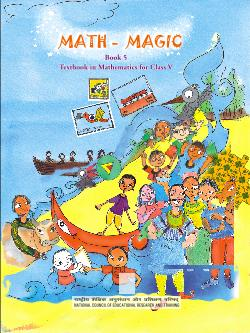## NCERT Solutions for Class 5 Maths Does It Look the Same

###### PRACTICE TIME:

1. Find out which letters in the English alphabet looks the same after a turn.

Ans. The letters in English alphabet which look the same after a turn are H, I, N, O, S, X and Z.

###### 2. Which of these English words reads the same on half a turn?

Ans. The English words MOW, SWIMS, SIS AND NOON read the same on half a turn.

NCERT Solutions for Class 5 Maths Does It Look the Same

###### 3. Give half a turn to the numbers from 0 to 9. Find which of them stills look the same.

Ans. The numbers 0, 1 and 8 look the same after giving a turn to them.

###### 4. Think of all 2, 3 and 4 digit numbers which look the same on half a turn.

Ans. All 2, 3 and 4 digit numbers which look the same on half a turn are:

2-digit numbers: 11, 88

3-digit numbers: 101, 111, 181, 808, 818, 888

4-digit numbers: 1001, 1111, 1881, 8008, 8118, 8888

NCERT Solutions for Class 5 Maths Does It Look the Same

###### 5. Which among the following pictures will look the same on half a turn?

Ans. The following pictures will look the same on half a turn.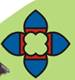###### 6. Have you ever seen a windmill? What is it used for?

Ans. Yes, I have seen a windmill. It is a mill turned by the wind. In it the wind turns a set of vanes mounted on a horizontal shaft, the rotation of which transmitted by gearing to working machinery. It is used for grinding corn, pumping water, street light and powering light industry.

NCERT Solutions for Class 5 Maths Does It Look the Same

###### 7.  Does you windmill look the same on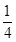of a turn?

Ans. Yes, the windmill look the same onof a turn.

###### 8. Does it look the same on half a turn? Discuss.

Ans. Sinceof a turn +of a turn means half of a turn. So it looks the same on half a turn also.

NCERT Solutions for Class 5 Maths Does It Look the Same

###### 9. Does the fan look the same onturn?

Ans. Yes, the fan looks the same onturn.

###### 10. Draw what the following shapes would look like onturn and half a turn.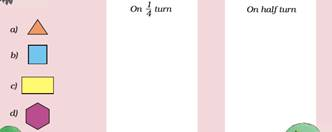Which of the above shapes do not look the same onturn? Which shapes do not look the same on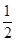a turn?

Ans.

 On 1/4th turn On half turn (a)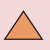(b)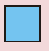(c)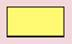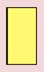(d)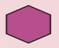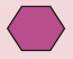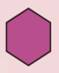Shapes (a), (c) and (d) do not look the same onturn. Shape (a) does not look the same ona turn.

NCERT Solutions for Class 5 Maths Does It Look the Same

###### 11. Which fan will look the same on a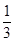turn?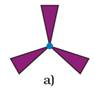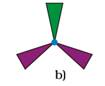Ans. Fan (a) will look the same on aturn.

###### 12. Look at the following shapes. Draw how they will look onandturn.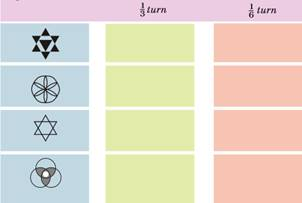Ans. The required shapes are drawn as under:

 1/3 turn 1/6 turn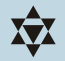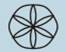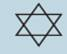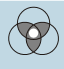NCERT Solutions for Class 5 Maths Does It Look the Same

###### 13. Look at the following shapes —

a) Find out which of these figures look the same onturn. Mark them with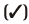.

b) Which are the ones that will not look the same afterturn? Mark them with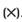.

c) Try and change the shapes below in such a way that they look the same onturn.

Ans. (a) Row 2nd:1st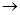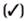, 2nd, 3rd(b) Row 1st: 1st, 2nd, 3rd(c)Given shapes are changed as under so that the may look the same onturn.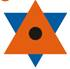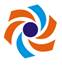###### 14.  Draw some shapes which will look the same afterturn.

Ans.  The following shapes will look the same afterturn.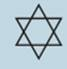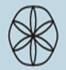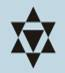NCERT Solutions for Class 5 Maths Does It Look the Same

###### 15. Draw some shapes which will look the same afterturn.

Ans. The following shapes will look the same afterturn.## NCERT Solutions for Class 5 Mathematics Chapter 5 Does It Look the Same

NCERT Solutions Class 5 Maths PDF (Download) Free from myCBSEguide app and myCBSEguide website. Ncert solution class 5 Maths includes text book solutions from Class 5 Maths Book . NCERT Solutions for CBSE Class 5 Maths have total 14 chapters. 5 Maths NCERT Solutions in PDF for free Download on our website. Ncert Maths class 5 solutions PDF and Maths ncert class 5 PDF solutions with latest modifications and as per the latest CBSE syllabus are only available in myCBSEguide.

## CBSE app for Students

To download NCERT Solutions for Class 5 Maths, Social Science Computer Science, Home Science, Hindi English, Maths Science do check myCBSEguide app or website. myCBSEguide provides sample papers with solution, test papers for chapter-wise practice, NCERT solutions, NCERT Exemplar solutions, quick revision notes for ready reference, CBSE guess papers and CBSE important question papers. Sample Paper all are made available through the best app for CBSE students and myCBSEguide website.Test Generator

Create question paper PDF and online tests with your own name & logo in minutes.myCBSEguide

Question Bank, Mock Tests, Exam Papers, NCERT Solutions, Sample Papers, Notes

### 3 thoughts on “NCERT Solutions for Class 5 Maths Does It Look the Same”

1. Nice

2. Very nice work

3. It is very good